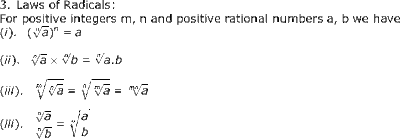## Tuesday, 12 June 2012

### CBSE - Class 9 - Maths - CH1 - Number Systems (Exercise 1.6)Al-Khwarizmi invented Algebra and Surds (src: wikipedia)

### Important points

1. If  a > 0, n and m are rational numbers, then
(i) am . an = am + n
(ii) (am)n = amn
(iii) am / an = am + n  , m >n
(iv) ambm = (ab)m
(v) a0 = 1

2.  If ‘a’ is a positive rational number and n is a positive integer such that, na  is rational number then
(i) na is called surd or radical
(ii) is called radical or radical sign
(iii) n is called order of radical
(iv) a is called radicand.
(v)  na = a (1/n)### Exercise 1.6

Q1: Find
(i) 641/2
(ii) 321/5
(iii) 1251/3

(i) 641/2 = (26)1/2 = 23 = 8
(ii) 321/5 = (25)1/5 =  2
(iii) 1251/3 = (53)1/5 = 5

Q2: Find
(i) 93/2
(ii) 322/5
(iii) 163/4

(iv) 125-1/3

(i) 93/2 = (32)3/2 = 33 = 27
(ii) 322/5 = (25)2/5 = 22 =4
(iii) 163/4 = (24)3/4 = 23 = 8
(iv) 125-1/3 = (53)-1/3 = 5-1 = 1/5 = 0.20

Q4: In the following equations determine whether a, b, c represent rational or irrational numbers.
(i)   a3 = 27
(ii)  b2 = 5
(iii) c2 = 0.09

(i)   a3 = 27 ⇒ a3 = 33 ⇒  a = 3          ... (rational number)
(ii)  b2 = 5   ⇒ b = √5                  ... (irrational number)
(iii) c2 = 0.09 = 9/100 ⇒ c2 = √(32/102) = 3/10 = 0.3     ... (rational number)

Q 5: What is successive magnification in number line representation?

Answer: The process of visualization of representation of numbers on the number line, through a magnifying glass is known as the process of successive magnification. The position of a real number with a terminating decimal expansion can be shown on the number line, by sufficient successive magnifications.

Q6: Give two irrational numbers so that their
(i) sum is not an irrational number.
(ii) difference is an irrational number.
(iii) product is an irrational number.
(iv) product is not an irrational number.

(i) sum is not an irrational number.
e.g. sum of √5  and (-√5) is 0 which is not irrational.

(ii) difference is an irrational number.
e.g. √5 - √3, the difference is irrational.

(iii) product is an irrational number.
e.g. √3 x √5 = √(15) which is an irrational number.

(iv) product is not an irrational number.
e.g. √(12) x √3 = √(36) = 6 which is a rational number.

Q7: Which one is greater √2 or 3√5 ?

LCM of 2 and 3 is 6. Above surds can be written as:
23/6and 52/6
⇒ 81/6and 251/6         (Now powers are same, we can compare radicands)
⇒ 251/6 > 81/6
or 51/3 > 21/2

Q8: If ax = b, by = c and cz = a then prove xyz = 1.

Answer: Given, ax = b, by = c and cz = a
⇒   (cz)x = b                  (Substitute value of a)
⇒   czx = b
⇒   (by)zx = b                 (Substituting value of c)
⇒   bxyz = b1
⇒   xyz = 1

Q9: If 2x-1 + 2x+1 = 320, find the value of x.

Answer: Given,  2x-1 + 2x+1 = 320
⇒   2-1.2x + 21.2x= 320
⇒   2x( 2-1 +  21) = 320
⇒   2x(5/2) = 320
⇒   2x = 320 x 2/5 = 128 = 27
⇒  x = 7

Q10: If ax-1 = bc, by-1 = ca and cz-1 = ab then prove that xy + yz + zx = xyz.

Answer: Given, ax-1 = bc, by-1 = ca and cz-1 = ab
ax-1 = bc
⇒  ax/a = bc
⇒  ax = abc
Similarly,  by = abc   and cxz= abc
Or we can write these terms as,

ax = abc  ⇒  a = (abc)1/x
by = abc  ⇒  b = (abc)1/y

cz = abc  ⇒  c = (abc)1/z

Multiplying above three equations give,

abc = (abc)1/x. (abc)1/y. (abc)1/z

⇒   abc = (abc)1/x + 1/y + 1/z
⇒  1 = 1/x + 1/y + 1/z
⇒  1 = (yz + xz + xy)/xyz
⇒  xyz = xy + yz + zx

Q11: If x, y are real numbers such that 3(x/y +1) - 3(x/y -1) = 24, the find the value of (x+y)/(x-y).

Answer: 3(x/y +1) - 3(x/y -1) = 24
⇒  31.3x/y - 3-1.3x/y = 24
⇒  3x/y(31 - 3-1) = 24
⇒  3x/y(9-1)/3 = 24
⇒  3x/y(8)/3 = 24
⇒  3x/y = (24).(3/8)
⇒  3x/y = ⇒  32
⇒  x/y = 2  = 2/1

Applying componendo and dividendo,

(x+y)/(x-y) = (2+1)/(2-1)

Thus (x+y)/(x-y) = 2

Q12: What are like surds and unlike surds?

Answer: Two surds ma and nb are like surds if m = n.
In case m ≠ n, the surds are unequal.1.bekar hai bekar ise kbhi bhi apna holiday homework na karna

2.bekar page hai ise bekar page mene aaj tak nahi dekha

3.Exercise 1.6 : Q 3 (ii) Answer = 1 by 3 to the power of 21.

4.This comment has been removed by the author.